Students can download 5th Maths Term 3 Chapter 2 Numbers InText Questions and Answers, Notes, Samacheer Kalvi 5th Maths Guide Pdf helps you to revise the complete Tamilnadu State Board New Syllabus, helps students complete homework assignments and to score high marks in board exams.

## Tamilnadu Samacheer Kalvi 5th Maths Solutions Term 3 Chapter 2 Numbers InText Questions

Try This (Text Book Page.No 15)

Write down the estimated value of numbers and find their sum. Also, find the difference of their sum.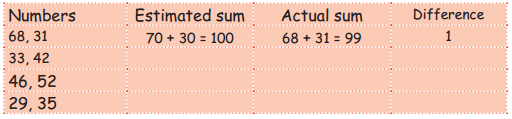Try This (Text Book Page.No 16)

Write down the estimated value of numbers and divide. Then find the difference between estimated value and actual value.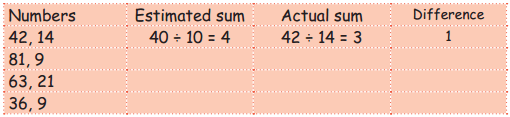Activity (Text Book Page.No 18)

A. Color the number’s row with the help of the following tips.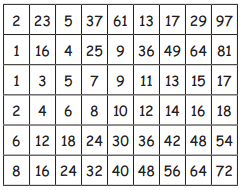1. Orange colour to the prime number row.
2. Don’t colour to the odd number row.
3. Blue colour to 6 in multiples row.
4. Orange colour to the square number row.
5. Dont colour to the even number row.
6. Blue colour to 8 in multiples row.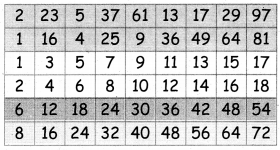B. Let us fill the number wheel
Question 1.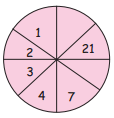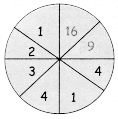Question 2.Question 3.C. Complete the circle using the given four basic operators to get the number 20.D. Fill in the blanks
Question 1.
5, 10, 15, ——, 25
20

Question 2.
30, 24, ——- ,126
18

Question 3.
7, 9, 11, ——-, ——-, 17
15Question 4.
1, 4, 9, ——-, 25
16

Question 5.
1, 4, 7, ——-,13, ——-, 19
10, 16

Question 1.
If BOOK is 43,
Then PEN is ———-
PEN = 16 + 5 + 14 = 35Question 2.
If SCHOOL is 1938151512,
Then CLASS is ———–
31211919

Question 3.
If BAG is 10,
Then BOOK is ———–
2 + 15 + 15 + 11 = 43

Question 4.
If LION is 50.
Then TIGER is ———–